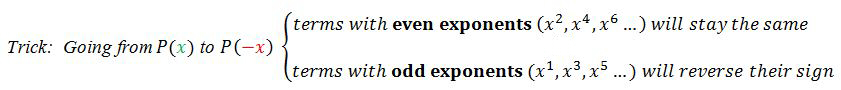Descartes' rule of signs

Descartes' rule of signs

Lessons

Descartes' Rule of Signs For a polynomial $P(x)$:
$\bullet$ the number of positive roots = the number of sign changes in $P(x)$, or less than the sign changes by a multiple of 2.
$\bullet$ the number of negative roots = the number of sign changes in $P(-x)$, or less than the sign changes by a multiple of 2.

Note: Before applying the Descartes' Rule of Signs, make sure to arrange the terms of the polynomial in descending order of exponents.• Introduction
Introduction to Descartes' Rule of Signs
a)
Fundamental Theorem of Algebra VS. Descartes' Rule of Signs

b)
Descartes' Rule of Signs – explained.
exercise: Use Descartes' Rule of Signs to determine the possible combinations of roots of:
$P(x)=2x^6-7x^5+x^4+5x^3-6x^2-10$

• 1.
Use Descartes' Rule of Signs to Determine the Number of Positive and Negative Roots
Use Descartes' Rule of Signs to determine the possible number of positive roots and negative roots:
a)
$P(x)=4x+9x^6-5x^2-10x^7+6x^3-8x^5-7x^4+3$

b)
$P(x)=x^4-5x^2-6x$ (note: NO constant term!!)

• 2.
Use the Rational Roots Theorem, together with Descartes' Rule of Signs, to Find Roots Effectively
Solve:
a)
$-3x^3+22x^2-37x+10=0$

b)
$-3x^3-5x-7x^2+2x^4-3=0$